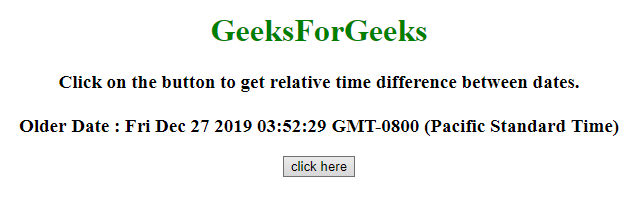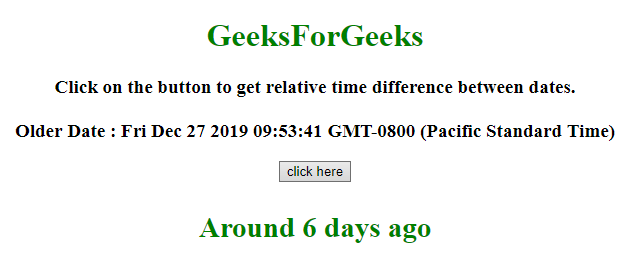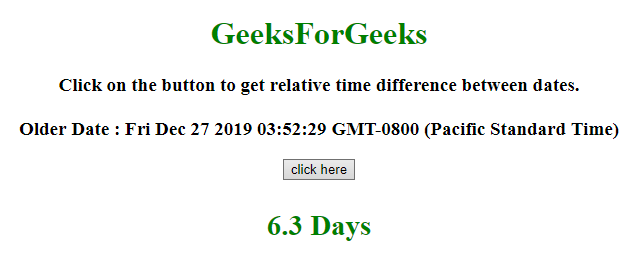# Get the relative timestamp difference between dates in JavaScript

• Last Updated : 28 Jan, 2020

Given the 2 JavaScript dates and the job is to get the relative time difference between them(eg.. 2 hours ago, 2.5 days ago, etc.) Here 2 approaches are discussed with the help of javaScript.

Approach 1:

Hey geek! The constant emerging technologies in the world of web development always keeps the excitement for this subject through the roof. But before you tackle the big projects, we suggest you start by learning the basics. Kickstart your web development journey by learning JS concepts with our JavaScript Course. Now at it's lowest price ever!

• Get the prevDate and currDate in a variable.
• Calculate the milliseconds in Minute, Hour, Day, Month and in an Year.
• Calculate the milliseconds difference between the prevDate and currDate.
• Compare these milliseconds with the milliseconds in Minute, Hour, Day, Month and in an year in this order.
• If the milliseconds are less than any of them, then calculate the respective Minutes, Hours, Days, Months and Years.

Example 1: This example implements the above approach.

 ` ` `<``html``> ` ` `  `<``head``> ` `    ``<``title``> ` `        ``Get the relative timestamp difference between dates in JavaScript. ` `    `` ` `    ``<``script` `src``= ` `"https://ajax.googleapis.com/ajax/libs/jquery/3.4.0/jquery.min.js"``> ` `    `` ` ` ` ` `  `<``body` `style``=``"text-align:center;"``> ` `    ``<``h1` `style``=``"color:green;"``>   ` `            ``GeeksForGeeks   ` `        `` ` `    ``<``p` `id``=``"GFG_UP"``> ` `    `` ` `    ``<``button` `onclick``=``"GFG_Fun();"``> ` `        ``click here ` `    `` ` `    ``<``p` `id``=``"GFG_DOWN"``> ` `    `` ` `    ``<``script``> ` `        ``var up = document.getElementById('GFG_UP'); ` `        ``var down = document.getElementById('GFG_DOWN'); ` `        ``var prevDate = new Date(); ` `        ``prevDate.setDate(prevDate.getDate() - 6); ` `        ``up.innerHTML = ` `            ``"Click on the button to get relative time difference between dates." + ` `            ``"<``br``><``br``>Older Date : " + prevDate; ` ` `  `        ``function timeDiff(curr, prev) { ` `            ``var ms_Min = 60 * 1000; // milliseconds in Minute ` `            ``var ms_Hour = ms_Min * 60; // milliseconds in Hour ` `            ``var ms_Day = ms_Hour * 24; // milliseconds in day ` `            ``var ms_Mon = ms_Day * 30; // milliseconds in Month ` `            ``var ms_Yr = ms_Day * 365; // milliseconds in Year ` `            ``var diff = curr - prev; //difference between dates. ` `            ``// If the diff is less then milliseconds in a minute ` `            ``if (diff < ``ms_Min``) { ` `                ``return Math.round(diff / 1000) + ' seconds ago'; ` ` `  `                ``// If the diff is less then milliseconds in a Hour ` `            ``} else if (diff < ms_Hour) { ` `                ``return Math.round(diff / ms_Min) + ' minutes ago'; ` ` `  `                ``// If the diff is less then milliseconds in a day ` `            ``} else if (diff < ms_Day) { ` `                ``return Math.round(diff / ms_Hour) + ' hours ago'; ` ` `  `                ``// If the diff is less then milliseconds in a Month ` `            ``} else if (diff < ms_Mon) { ` `                ``return 'Around ' + Math.round(diff / ms_Day) + ' days ago'; ` ` `  `                ``// If the diff is less then milliseconds in a year ` `            ``} else if (diff < ms_Yr) { ` `                ``return 'Around ' + Math.round(diff / ms_Mon) + ' months ago'; ` `            ``} else { ` `                ``return 'Around ' + Math.round(diff / ms_Yr) + ' years ago'; ` `            ``} ` `        ``} ` ` `  `        ``function GFG_Fun() { ` `            ``\$('#GFG_DOWN').html(timeDiff(new Date(), prevDate)); ` `        ``} ` `    `` ` ` ` ` `  ` `

Output:

• Before clicking on the button:• After clicking on the button:Approach 2:

• Get the prevDate and currDate in a variable.
• Calculate the seconds, Minutes, Hours and Days difference between the dates.
• Compare these parameters with the 60 seconds, 60 Minutes, 24 Hours, Day in this order.
• If any of those condition satisfies then return the respective parameter.

Example 2: This example implements the above approach.

 ` ` `<``html``> ` ` `  `<``head``> ` `    ``<``title``> ` `        ``Get the relative timestamp difference ` `      ``between dates in JavaScript. ` `    `` ` `    ``<``script` `src``= ` `"https://ajax.googleapis.com/ajax/libs/jquery/3.4.0/jquery.min.js"``> ` `    `` ` ` ` ` `  `<``body` `style``=``"text-align:center;"``> ` `    ``<``h1` `style``=``"color:green;"``>   ` `            ``GeeksForGeeks   ` `        `` ` `    ``<``p` `id``=``"GFG_UP"``> ` `    `` ` `    ``<``button` `onclick``=``"GFG_Fun();"``> ` `        ``click here ` `    `` ` `    ``<``p` `id``=``"GFG_DOWN"``> ` `    `` ` `    ``<``script``> ` `        ``var up = document.getElementById('GFG_UP'); ` `        ``var down = document.getElementById('GFG_DOWN'); ` `        ``var prevDate = new Date(); ` `        ``prevDate.setDate(prevDate.getDate() - 6); ` `        ``prevDate.setHours(prevDate.getHours() - 6); ` `        ``up.innerHTML = ` `            ``"Click on the button to get relative time " + ` `            ``"difference between dates.<``br``><``br``>Older Date : " + prevDate; ` ` `  `        ``function conversion(ms) { ` ` `  `            ``// Calculating Seconds ` `            ``var sec = (ms / 1000).toFixed(1); ` ` `  `            ``// Calculating Minutes ` `            ``var min = (ms / (1000 * 60)).toFixed(1); ` ` `  `            ``// Calculating hours  ` `            ``var hrs = (ms / (1000 * 60 * 60)).toFixed(1); ` ` `  `            ``// Calculating days ` `            ``var days = (ms / (1000 * 60 * 60 * 24)).toFixed(1); ` `            ``if (sec < ``60``) { ` `                ``return sec + " Sec"; ` `            ``} else if (min < 60) { ` `                ``return min + " Min"; ` `            ``} else if (hrs < 24) { ` `                ``return hrs + " Hrs"; ` `            ``} else { ` `                ``return days + " Days" ` `            ``} ` `        ``} ` ` `  `        ``function GFG_Fun() { ` `            ``var ``date` `= ``new` `Date(); ` `\$('#GFG_DOWN').html(conversion(date.getTime() - prevDate.getTime())); ` `        ``} ` `    `` ` ` ` ` `  ` `

Output:

• Before clicking on the button:• After clicking on the button:My Personal Notes arrow_drop_up
Recommended Articles
Page :